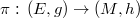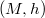﻿

### Biharmonic Hermitian vector bundles over compact Kähler manifolds and compact Einstein Riemannian manifolds

#### Abstract

We show, for every Hermitian vector bundle$\pi:\,(E,g)\rightarrow (M,h)$ over a compact Kähler Einstein manifold$(M,h)$, if the projection$\pi$ is biharmonic, then it is harmonic. On a biharmonic Hermitian vector bundle over a compact Riemannian manifold with positive Ricci curvature, we show a new estimate of the first eigenvalue of the Laplacian.

DOI Code: 10.1285/i15900932v39n2p95

Keywords: biharmonic maps; harmonic maps; Kähler Einstein manifolds; Hermitian vector bundles

Full Text: PDF